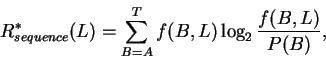>Next: (d) Programs and Computers Up: 2. Materials and Methods Previous: (b) Formula for Rfrequency

## (c) Skewed Genomes8

This paper considers the relationship between Rsequence and Rfrequency. For restriction enzymes cutting genomes with equal numbers of the four bases randomly distributed, Rsequence and Rfrequency are equal. For example, one commonly assumes that HaeIII (GGCC; Roberts, 1983; Rsequence = 8 bits) cuts once in 256 bases ( Rfrequency = 8 bits). This is not true for "skewed" genomes, in which the frequencies of each base are significantly unequal. For example, in a genome like that of bacteriophage T4 which is two-thirds A-T, Rsequence for any tetramer is 7.7 bits. Yet GGCC should occur once in every 1296 bases ( ( 1 / 6 )4; Rfrequency = 10.3 bits) and conversely AATT should occur once in every 81 bases ( ( 1 / 3 )4; Rfrequency = 6.3 bits). An alternative formula,(10)

matchs Rfrequency in examples of this type. When the genomes are equiprobable, as they are in this paper, the two Rsequence formulas give the same values. We suggest that both be tried for sites in skewed genomes.Next: (d) Programs and Computers Up: 2. Materials and Methods Previous: (b) Formula for Rfrequency
Tom Schneider
2002-10-16